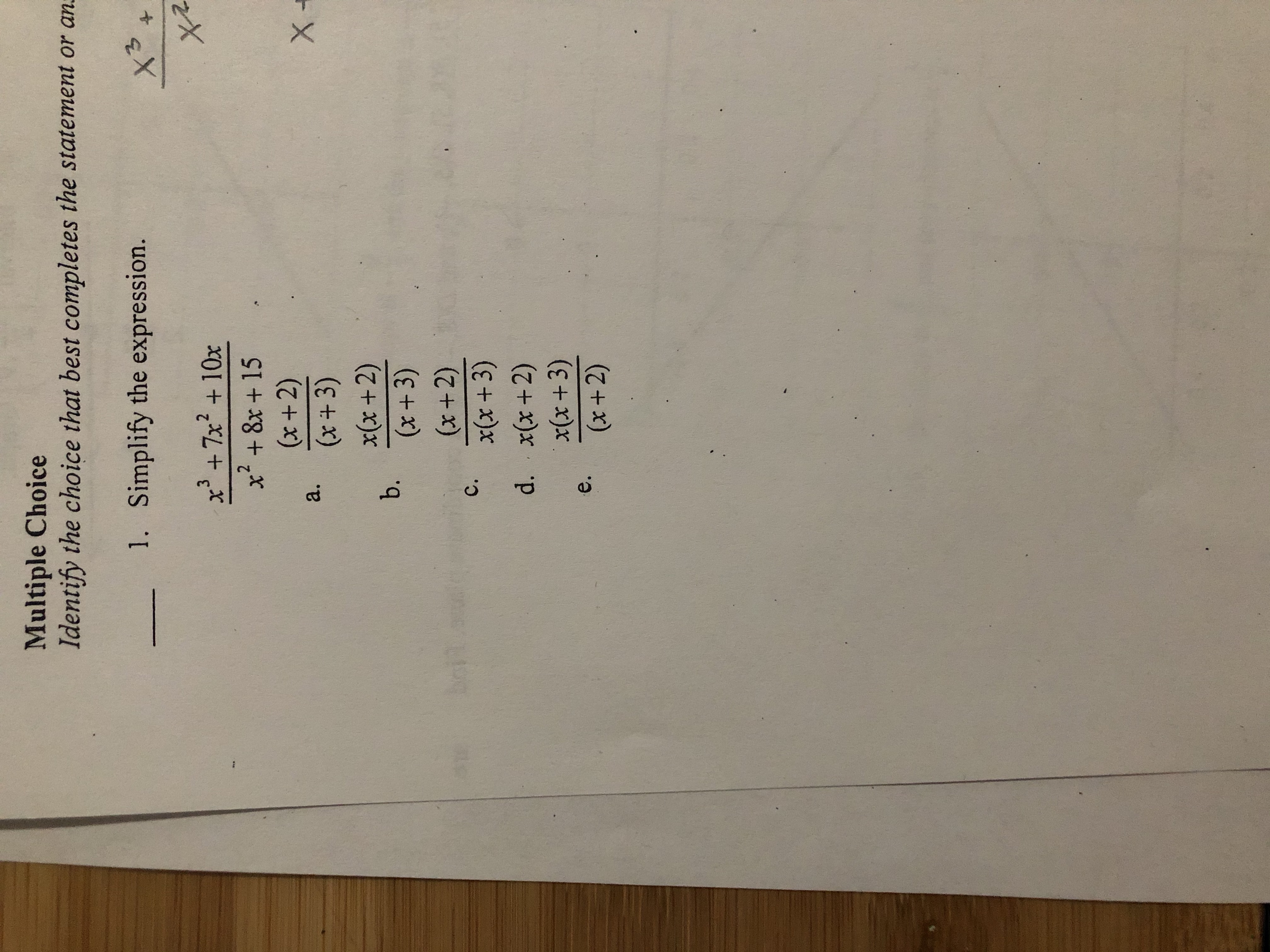# Multiple ChoiceIdentify the choice that best completes the statement or an:1.Simplify the expression.132.x' +7x +10xx+ 8x + 15(x+2)(x+ 3)x(x +2)b.(x+3)(x+2)C.x(x+3)d. x(x+2)x(x+3)e.(x+2)a.

Question
2 viewshelp_outlineImage TranscriptioncloseMultiple Choice Identify the choice that best completes the statement or an: 1. Simplify the expression. 13 2. x' +7x +10x x+ 8x + 15 (x+2) (x+ 3) x(x +2) b. (x+3) (x+2) C. x(x+3) d. x(x+2) x(x+3) e. (x+2) a. fullscreen
check_circle

Step 1

The given expres...

### Want to see the full answer?

See Solution

#### Want to see this answer and more?

Solutions are written by subject experts who are available 24/7. Questions are typically answered within 1 hour.*

See Solution
*Response times may vary by subject and question.
Tagged in

### Other